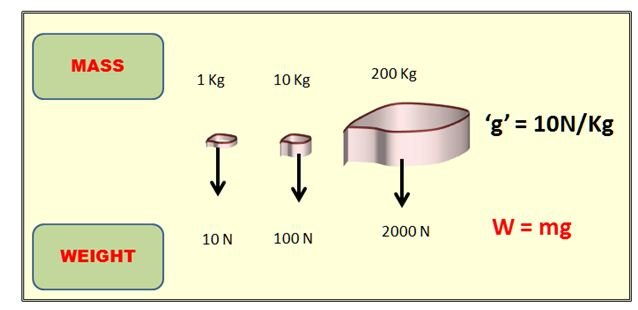# Definition Of Weight

## Definition Of Weight

"The force with which body is attracted towards earth."
Weight is the one more name for Earth’s gravitation force on an object.
Spring balance is used to measure the weight of the object.

The weight of the object is equal to product of mass and gravitational field strength at that particular place.
The gravitational field strength (g) changes from one place to another place.
On the surface of the earth, an object of mass 1 kg has a weight of 9.8N (For our convenience we consider 10N for calculation purpose).#### Worked Example:

At a point on the surface of the Earth, the gravitational field strength is 10N/Kg.
Which pairs of values for mass and weight are correct for an object placed at this position?

 mass /kg weight/N A 10 10 B 20 2 C 50 100 D 2 20

#### Solutions

Step 1: Gravitational field strength is 10 N/kg (given).
Step 2: Weight = mass x gravitational field strength: gravitational field strength = Weight /mass.
Step 3: A- 10/10 = 1 but ≠10
B- 2/20 = 0.2 but ≠ 10
C- 100/50 = 2 but ≠10;
D- 20/2 = 10 = gravitational field strength (given). Therefore D is the correct answer.

#### Worked Example

What is the weight of a person on the surface of the moon, if his mass on earth is 100Kg?
Gravitational field strength on the moon is 1.6N/kg.

A. 100 N
B. 200 N
C. 160 N
D. 16 N